# Multiplication & Convolution on a Linear Array

Tom Kelliher, CS 315

Apr. 14, 1999

### From Last Time

Linear array multiplication example, run time calculation.

### Outline

1. Returning to the run time.

2. Three optimizations.

3. Convolution, connections.

### Coming Up

Shuffle-exchange networks.

# Multiplication on a Linear Array

1. Run time, efficiency, work.

What did we forget about in the run time calculation?

## Improvement 1

2. Reworked example.

3. Changes to run time, efficiency, work?

## Improvement 2

1. All input from one side of array.

2. Reworked example.

3. Changes to run time, efficiency, work?

## Improvement 3

Using the original array to perform two multiplications in parallel.

## Optimality

Show that this algorithm is optimal for a linear array.

# Connecting to Convolution

The convolution of the N-vectors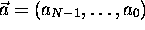and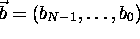is the 2N - 1 vector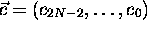where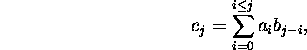where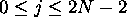.

• How does this relate to integer multiplication?

• How does this relate to polynomial multiplication?

• Use in signal processing.

Thomas P. Kelliher
Wed Apr 14 10:10:05 EDT 1999
Tom Kelliher